﻿ Applying Differentiated Teaching Method in Teaching Mathematics in High Schools in VietnamPublications are Open
Access in this journal
Article Versions
Export Article
• Normal Style
• MLA Style
• APA Style
• Chicago Style
Research Article
Open Access Peer-reviewed

### Applying Differentiated Teaching Method in Teaching Mathematics in High Schools in Vietnam

Do Thi Hong Minh
American Journal of Educational Research. 2018, 6(5), 532-538. DOI: 10.12691/education-6-5-26
Received February 16, 2018; Revised May 03, 2018; Accepted May 05, 2018

### Abstract

With the continuous development of society and education, it is possible to realize that differentiated teaching is an indispensable trend and objective requirement. Because of social requirements for human resource training, there are both similarities in the personality of workers, and differences in the workers’ level of development, the tendency as well as talent in the same society. Students, who are at the same age, usually have both similarities, and differences in perceptions, thinking, talents, strengths, conditions, circumstances, family backgrounds... In this article, the author presents an overview of differentiated teaching method: Including the nature, goals, processes, forms, and some teaching techniques used in, how to assess in differential teaching and what teachers should do in an differentiated classrooms as well. The author also presents the level hierarchy of activities in mathematics teaching, which is a basis for the design of differential lectures and a teaching phase applying differentiated teaching in teaching Mathematics in high school.

### 1. Introduction

Differential teaching has been studied and used extensively in many countries around the world. In the book Building Teachers: A Constructivist Approach to Introducing Education 1, David and Kimberly point out that it is effective to put different learning styles and minds into a lesson in differential teaching method. There will be a number of teaching strategies corresponding to each feature to help recognize that feature and there are several common patterns that connect all or most of the distinctive features. The authors, David and Kimberly, summarize four main elements to identify differentiated classes that is organized in differentiated ways, those are: Doing exercises in small groups with classmates; Working with teachers individually or in small groups; Spending different amounts of time in a task to help learners learn well; Working with different materials to help learners learn well.

In the Leading and Managing A Differentiated Classroom 2 and Differentiation of Instruction in the Elementary Grades 3, Carole Ann Tomlinson and Marcia Imbeau have argued that differential teaching strategies require teachers to have to point out the goal of learning is rooted from standards of content, but implementing is skillful to ensure that every student is taken part in and understands their lessons.

In Vietnam, the main idea of differentiated teaching is clearly mentioned in Nguyen Ba Kim's Method of Teaching Mathematics 4. The author argues that it is necessary to combine between "mass education" and "key education”, as well as encouraging maximum and optimal development of individual capabilities. Many recent articles that have mentioned the differential teaching at school 5, 6, 7, 8, 9, 10, 11, 12, 13..., discuss the real situation of differential teaching and solutions to the effective implementation of differential teaching dissemination to meet current curriculum and textbook requirements 14.

One of the problems of traditional teaching is the lack of diversity in the classroom. The curriculum and teaching methods still apply to the majority. This raises a question: How to maximize the individual ability of each learner? And one of the pedagogical solutions offered is a differential teaching organization. Characteristics of differential teaching are to detect and compensate for learning gaps, to motivate learning, to transform the passion in life into motivation in learning.

For a credit hour teaching Mathematics at high school, how to teach to ensure three requirements: Improving knowledge for the advanced students; equipping with basic knowledge for the average students; and filling up gaps for struggling students? In my opinion, it is possible to apply in a mathematics class to all students in the class with appropriate questioning and exercise which are suitable for level of students in class. It is necessary to take the general level of development of the students in the class as a foundation, add some contents and differentiating method to help the better students reaches the advanced requirements on the basis of achieves the basic requirements. Using differentiating method raises students from risk level upto common level. By using differential teaching methods in the classroom, students will be able to develop their abilities, acquire knowledge actively and creatively depending on the level of awareness of each student.

This article hopes to give readers an overview of differential teaching and use this teaching method in teaching Mathematics in high school.

### 2. Content

2.1. Differential Teaching
2.1.1. The Essence of Differentiated Teaching

Differential teaching is a teaching strategy that based on the teacher's perceptions of the learner’s individual needs. Differential teaching comes from the dialectics of unification and distinction, from the requirement to ensure the full realization of all teaching purposes while encouraging maximum and best development of individual capabilities 4.

In fact, students in class have many differences of views and abilities. Therefore, teaching methods of teachers need to be divided according to learners. Differential teaching strategies require teachers to clarify learning goals that proceed from standards of content and are implemented skillfully to ensure that all students participate in and understand the lesson. The nature of the differential teaching process is adjustments of the content of knowledge to meet the needs, ability and experience of students.

2.1.2. The Goal of Differential Teaching

The main idea of differential teaching is that taking the general level of development of the students in the class as the foundation; trying to bring the weak to the medium; Seeking to bring the good, and quite good students to meet the advanced on the basis of achieving the basic requirements. Therefore, the principle of differential teaching is that the teacher must admit that the learners are different; pay more attention to quality than quantity; Focus on learners, learning is appropriate and exciting; Merge teaching the whole class with group and individual instructions .

As such, it is possible to see that differentiated learning makes the teaching process and system more adaptable to the individual learner, with the characteristics of the target group to ensure learning quality and to meet educational goals, needs and social benefits effectively.

2.1.3. Differential Teaching Process

The differential teaching process consists of 3 steps:

- Step 1: Classify students by the levels of awareness and needs:

Teachers must classify students into different groups. To do so, the teacher needs to perform initial assessments (official or unofficial) at the time near the lesson. And then, teachers will then determine the levels of awareness, the student’s interests in their learning.

- Step 2: Build and implement the differential teaching plan:

Based on information on the cognitive level of the students, and combination with the standards of knowledge, skills and attitudes, teachers set teaching goals for each student, select teaching contents and carry out the teaching process in the direction of division.

- Step 3: Assess and Review:

Teachers conduct official and unofficial assessments from that point to draw necessary experiences and make adjustments and additions timely to improve the efficiency of the next teaching and learning process.

2.1.4. Forms of Differential Teaching

Differential teaching is held in various forms:

- Differentiation with interest (based on the student's interest in learning to organize learners to explore knowledge).

- Differentiation with perception (taking the tempo discrimination as a differentiated basis. The pace is calculated by the amount of time that moves from activity to activity, from task to task).

- Differentiation of school time according to the learning capacity (based on the learners' actual learning level to have suitable pedagogical impacts to learners. Based on the good or average or weak level of learner, the teacher assigns learner the corresponding tasks).

- Differentiation of school time according to learners' learning motivations and benefits (for groups of students who have high learning needs, they need to identify higher learning tasks and put more contents and learning materials so that they are able to teach themselves. For groups of students who have low learning needs, the distribution of teaching must pay attention to tasks, basic contents and add practical problems to help learners feel very highly enthusiastic in their learning).

In the form of differentiated teaching, teachers plan and compose lecture so as to integrate as many teaching strategies as possible to aim at recognizing the differences of the students in the class. Differential teaching includes the following: Adjust content of the lesson to meet students' abilities, experiences, and interests; Bring out variety of ways to achieve the objectives of the lessons; Allow students to prove their knowledge in meaningful ways; Allow for diversity in the learning environment that based on the needs of each student; It is not required that teachers build individualized teaching plan for each student. Instead, this method requires teachers to look for types of demand and then subgroup students with similar needs or interests so that teachers can meet the needs of each group.

2.1.5. Teaching Techniques Used in Differentiated Teaching

To teach differentiated learning, teachers often use the following skills:

- Skills to assess and classify students: Teachers can base on one of the following factors: the standard output of the subject, cognitive level, cognitive pace, learning excitement, and student’s learning style. From that point, the teacher builds teaching goals at different levels and selects the appropriate form of organization and teaching methods.

- Skills to design and use of learning resources in the teaching process: Teachers need to be well prepared about the teaching environment, teaching conditions and facilities to aim at assisting self-learning and self-studying activities of students.

- Skills to build the contents of differentiated teaching: Deepening the basic knowledge, developing subject’s curriculums and lesson’s plans; setting suitable cognitive tasks for each student’s objective; choosing teaching contents that correspond with developing students' strong points.

- Skills to combine teaching methods and teaching forms: Teachers should use various forms of teaching organization as the whole class, small groups, individuals that depend on the learning conditions. Using teaching methods to ensure the principles the learner self-completes their cognitive tasks with the organization and guidance of the teacher. Thus, learners exercise and develop logical thinking, critical thinking, and creative thinking through the activities of analysis, synthesis, comparison and application.

- Skills to manage classroom and create differential teaching environment:

+ Allocate reasonable time: Teacher should consider and allocate time flexible for each activity to suit the students. One of the most effective ways to distribute time is that to use the 'anchor' activity. This activity allows all students to be self-motivated to move from one activity to another activity when they have completed their assigned tasks.

+ Calculate carefully how assignments exercise and tasks for students:

The first way is designing and bringing out learning tasks for individual student or groups of students through learning cards or tickets.

The second way is that the teacher can assign a task to some responsible students and these students will inform and exchange that task with their group mates. Teachers should carefully consider these tasks and anticipate the mistakes that students often make, and the psychological difficulties that students must overcome as well as the problems that may arise when part of the contents of task requires students on the move to control time. Later, the teachers choose how to advise and guide students to complete the task of learning.

+ Exploit of support activities of teachers and classmates;

+ Enhance the sense of responsibility of each student in learning activities, self-study, cooperate with teachers, cooperate with friends in the learning process.

2.1.6. The Works that the Teacher has to do during Differentiated School Time

Before each lesson, the teacher should review the results from the previous session to determine if any student needs to be transferred from the lower level to the higher level, which content takes a lot of time to study, what parts of content that students make many mistakes? Next, teachers check the contents of the new lesson to determine if it is necessary to reduce any content or add any activities or not? Finally, the teacher reads the lesson in the textbook and schedules additional activities during the lesson (exercises, games, hands-on activities, etc.).

Particularly, in the differentiated teaching, it is necessary to conform the four-step process, including: survey and survey of students before teaching; Planning teaching schedule, composing lesson to base on analyzing from the needs of students; During teaching hours, teachers must combine teaching methods, select the teaching forms that are suitable for the lesson objectives; Examine and evaluate students’ progress during the process of teaching.

2.1.7. Evaluation in Differentiated Teaching

In order to teach differentiated learning, teachers must have the assessment skills to assess students’ learning results in the direction of differentiation. So, from the designing of subject outline, the teachers must establish the target matrix of subject, lesson and build questionnaire banks to evaluate respectively. To ensure equity in differential teaching assessments, teachers must have the capacity to assess regular, progressive in each small-phase assessments and pay particular attention to assess the progress of each student. If it is based on the studying ability of students to required implementing the tests have the differentiated difficulties in the requirements of the tests. If it is based on the learning style and excitement of students, the teacher must design multiple test forms for each group. It is disadvantage for students who have characteristic introverted learning intelligence, but teachers require assessment by team interaction. Conversely, students who tend to like movement and practice are unlikely to take a written test. This is not an easy requirement for the teacher. This is a very important step so that differential teaching comes to success.

Teachers must constantly adjust and complete their differentiated teaching activities. This is considered to be the last stage of the differential teaching activity as well as the preparation for the next differential teaching strategy. When results appear in the teacher's assessment, the teacher must analyze the causes of the achievement and failures in the results of the differentiated teaching. It is necessary to re-examine the stages of the differential teaching activities, to adjust and improve the differential teaching ideas of their own gradually, to use the evaluation results to adjust the teaching process and control the next learning process of students.

2.2. Level Hierarchy of Activities in Mathematics

In differentiated mathematics teaching, level hierarchy of activities is the basis for the design differentiated lectures and controls the teaching process.

It is important for the teachers to determine the levels of required instruction in the activities that students must achieve at the end of each session or at intermediate stage. Here, the term "level" and the term "level hierarchy" can be understood both in the sense of "macro" has the sense of "micro". In macro sense, I say to the various stages of the entire period of studying in high school, a class or a certain educational level. In micro level, the level of activities is defined as the required level in a short period of time or maybe just in a lesson.

In mathematics differentiated teaching in high school, level hierarchy of activities suits each group of students is extremely necessary. The level hierarchy of activities is able to use to implement internal differentiated teaching in the way that allows students in different skill levels to perform activities simultaneously with the same content but at different levels of demand. While we are applying mathematics differentiated teaching, if level hierarchy of activities which is understood by “micro” sense is not showed in the curricula and textbooks, Teachers will need knowing how to perform level hierarchy of activities flexibility. According to Nguyen Ba Kim 4, whether in the macro or micro sense, the level hierarchy of activities is able to base on the following:

2.2.1. Complexity of the Activity’s Objects

The more complex object is, the more difficult it performs. Therefore, it is possible to base on the complexity of the object to grade activities.

Example: When the teacher teach about the solution and argue the basic trigonometric equation, the teacher can give the differential exercises which base on the complexity of the activity’s objects as following:

Solve the following equations: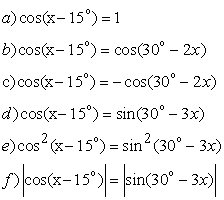The a, b are basic, prototype equations, which can be given to the weak students;

The c, d are two equations that are close to the basic form, so students need to change the equations into basic form by modification: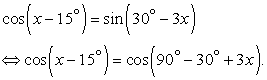And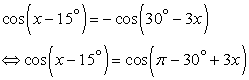so this equations can be given the average students;

Question d, e are two equations that have other forms, they can be transformed into the basic form by squaring root and subtracting absolute values as following: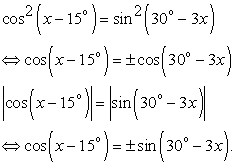Therefore, these questions can be for advanced students.

2.2.2. Abstraction, Generalization of the Object

The more abstract and generalized objects are, the higher performance’s requirements are, the activities at high level will be more general than activities at low level. Therefore, the levels of the object’s abstraction and generalization is the basis of level hierarchy of activities.

Example: When The teachers teach how to solve the trigonometric equations which have the form: a(sinx + cosx) + bsinx.cosx + c = 0

In this problem, if the teacher asks the whole class to think of ways to solve this problem, only a few students will be able to do it. They will be very confused and do not know where to start, because it hasn’t been available familiar form to implement the algorithm yet. Therefore, the teacher can give the exercises which have level hierarchy of activities based on the object's abstraction and generalization through suggesting students to do sub-questions so that all students in the class can approach to the problem as following:

For sinx + cosx = t (1)

a) Solve the equation with t = 1

b) Calculate the expression sinx.cosx by t

c) Solve the equation: 2(sinx + cosx) - 3sinxcosx - 2 = 0

d) Generalize the solution of the equations that have the form: a(sinx + cosx) + bsinx.cosx+c = 0 from the a, b, c above.

The a is a familiar problem; students will solve this equation by using the formula: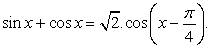The question b: To calculate the sinx.cosx expression by t, they will square two sides of equation (1) to draw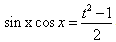. Therefore, a and b are able to use for weak students.

The question c: In order to solve this equation, students will see suggestion of the solutions of the a and b above, they will put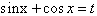(with the condition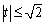because the a shows that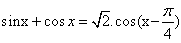). And then, we replace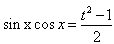to have familiar quadratic equation with unknown t: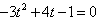. So, b, c are able to intend for the average student.

The question d: After students have solved the questions a, b, c, for advanced students will generalize by themselves the solution of the equation that has general form. So the c, d are able for advanced students.

2.2.3. Contents of the Activity

The contents of the activity are almost knowledge which have related to the activity and other conditions of the activity. The more activity’s contents are, the more difficult activity’s performance is, so the activity’s contents are also the basis of level hierarchy of activities.

Example: level hierarchy of activities that is based on the activity’s contents is expressed clearly in the goal of each lesson in differentiated teaching, such as the lesson in basic trigonometric equations:

- For the weak students: know and understand the solving algorithm of the basic trigonometric equations;

- For the average students: apart from knowing and understanding the algorithm of the basic trigonometric equations, they know how to transform non-basic trigonometric equations into basic equations to solve.

- For advanced and excellent students: apart from the goals as for the average students, he or she knows how to solve and argue the basic trigonometric equation with parameters, or know to applying the basic trigonometric equations to some other math forms.

2.2.4. Complexity of Activities

A complex activity consists of many component activities. Increasing these components also means improving the request for the activities.

Example, when teacher assign students the exercises in the teaching of the trigonometric equations that have the form "asinx + bcosx = c", the teacher can give exercises which have level hierarchy of activities to base on the complexity of activities as following:

For the equation: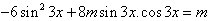a) Solve the equation when m = 1

b) With m = 1, find the solutions of the equation to satisfy: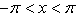c) Find conditions of m to this equation has solution.

In this exercise, the problem’s complexity increases in each question a, b, c.

For the a: When m = 1, the equation will be transformed into the basic form: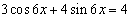For the question b: To solve this question, students must solve the question a to find the solutions of this equation and then find the solutions to satisfy: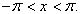For the question c: To solve this problem, students need to transform the equation into the common form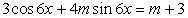and apply the general equation’s condition to the equation has solution, that is: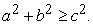Thus, in the differentiated teaching of this content, teachers can assign tasks to the groups as following: Exercise a can give to weak students; For the average students, they are able to do the exercises a, b; For advanced and intelligent students, the teacher asked to do the questions b, c.

2.2.5. Quality of Activities

The quality of activities usually is independence or proficiency that can also be used as a basis for level hierarchy of activities.

Example: In the lesson of reviewing trigonometric equations, teachers can give many exercises that have the same forms to train students’ proficiency in solving basic or common trigonometric equations for weak and average students, such as:

Solve the equations as following: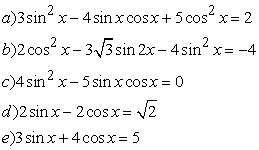At the same time, for advanced students, they have had the skills to solve trigonometric equations; teachers can give some advanced exercises to improve their independence and creativity, as following: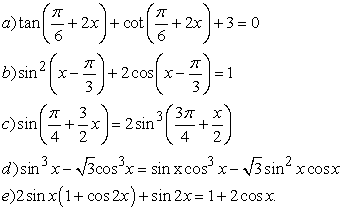2.2.6. Combine Multiple Aspects as a Basis of Level Hierarchy of Activities

Example: In the examples (2.2.4) above, there is the combination of the complexity of activities and complexity of the activity’s objects as a basis of level hierarchy of activities.

2.3. Example of the Differential Teaching Situation in Teaching Mathematics at High School

In teaching Mathematics, there have contents about equations and equivalent equations transformation (grade 10 at High School). For an unequal perception class, to fill the gap of knowledge for the weak students, equip the average students with standardized knowledge and improve their knowledge for good and excellent students, teachers can carry out the differential teaching as follows:

Subject: Equivalent conversion and irrational equation solving.

2.2.1. Target

- Common objective:

+ Students apply the concept of equivalent equations to solve specific problems.

+ Students identify and correct common mistakes that are about equivalent transformation.

- For the weak students: Know how to transform equivalent equations and apply them to solving basic equations;

- For the average students: Complete the common goals;

- For advanced students: Achieving common goals; Flexible use of learned knowledge to solve more complex equations.

2.2.2. Steps to Proceed

Activity 1: Teachers divide students into small groups (weak, average, advanced) based on the level of the students’ awareness of as in the previous session. However, the teachers can base on the results of the previous study; the teacher has shifted of some students who make much progress in their studies from low levels to higher levels.

Activity 2: Teachers repeat the some of the knowledge about equivalent transformative that have been applied in solving irrational equations or inequations.

When solving an equation or an inequation containing unknown in the root, we must perform some equivalent transformations to bring it to an equation or inequation that doesn’t contain unknown in the square root. During the transformations process, note:

+ Mention the determined conditions of the equation and state the condition of the root (if available).

+ Just square both sides of the equation when both sides are non-negative.

+ Add up those conditions with the new equation or inequation we get, which has a system of equations equivalent to the given equation (namely, the equation and the system of equations have the same set of the equations’ roots).

This is the basic, common method that is applied for many irrational equations. When solving the irrational equation, firstly, we find condition (if available) to the equation means, and then try to eliminate the root. In order to do that, we often use the transformations equation to convert that equation into the equivalent equation by exponential the two sides of the equation to reduce the root, but when choosing the roots, it is important to note the limited condition of the root to reject the roots that isn’t suitable.

When solving irrational equation, students do not often distinguish them when the transformation is equivalent, when it is the consequence, that of the cause to appear alien roots. Therefore, it is important for the students to note the following:

With the exponent of even degree of two sides of an equation, if you want the equivalent equation, you have to set the condition so that two sides of equations are non-negative. So when solving the equation with the roots, we just need to test the condition without trying that roots into the original equation.

And when the exponent of even degree in both sides of the equation without conditions, new equation is only the consequent equation. So when the finding the roots of the last equation, we have to test again into the original equation to reject alien roots.

+ When the exponent of odd degree of two sides of an equation we always have the equivalent equations.

Activity 3: Give the differentiated exercises.

Exercise: Use equivalent transformations and theoretical basis to solve equations.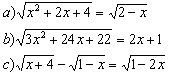Activity 4: Group discussion

- Group 1 (weak): Solve (a) equation

- Group 2 (average): Solve (b) equation

- Group 3 (pretty good): Solve (c) equation, from which writes the roots of the equation: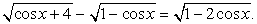At the same time generalize for the problems.

Activity 5: The groups present their exercises

Group 1: Present how to solve (a) equation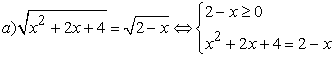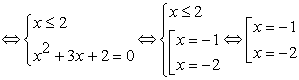The roots of the equation are x = -1 and x = - 2

Group 3: Generalize how to solve of the equations in the form: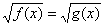*Solution: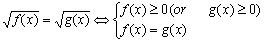Group 2: Present how to solve (b) equation.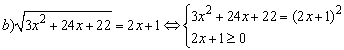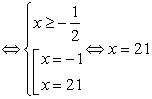The root of the given equation is x = 21.

Group 3: Generalization: The equations have the form: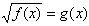. This is a basic form of irrational equation, which is usually solved by the two-side exponentiation method.

*Solution: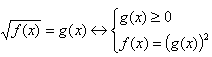Group 3: Present how to solve (c) equation.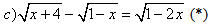Conditions: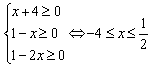With above conditions, the given equation is equivalent to the following equation: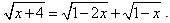Noticed two sides of the equations are non-negative, squared two sides of the equation, we get: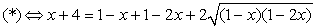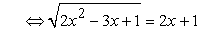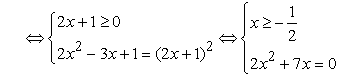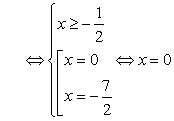The root of the given equation is x = 0 (satisfy condition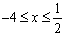)

Write the roots of the equation: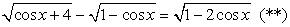By setting the hidden sub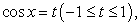the form of the (**) equation is the same as the (*) equation, so the (**) equation has the solution: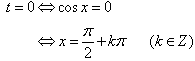Group 3: Generalization: The equations have the form: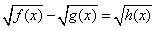(1)

Solution: Setting conditions: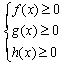Transform the equation to which two sides of this equation are non-negative: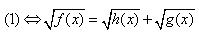Square two sides of the equation, we get: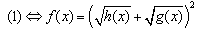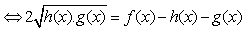(2)

This is an equation which has the form: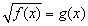shown in b)

After completing the (2) equation, it is necessary to compare the roots of the (2) equation with the conditions set above to reject alien roots.

Activity 6: The teacher summarizes the knowledge of the irrational equation and reviews and assesses the presentation and the progress of the groups.

The teacher gives differentiated exercises so that different students can conduct different activities at different levels. The teacher can differentiate the requirements by using a graduated exercise circuit, assigns good students exercises that have higher-level activate than other students. Or even in an exercise, the teacher can conduct differential teaching if that exercise ensures the required activity for all three groups of students, and the differentiated exercise aims to:

The average and weak students often show to have no basic knowledge and skills, reveals serious mistakes and gaps in knowledge.

Because the students of higher level have good capacity to study mathematics, they tend to prefer solving many mathematical problems, solving difficult math problems and mathematical problems, that require creative thinking, but they disregard theoretical learning, and normal math problems. They are subjective and neglected. That is the cause leading to mistakes in the mathematical solutions.

Based on these results, it is necessary for weak students to fill holes of knowledge, equip standard knowledge for average students and improve knowledge for more advanced students.

### 3. Conclusion

Differential teaching is an indispensable trend in today's society. Differential teaching is based on the actual demand of class. There is always the difference in the levels of awareness of each member, ensuring good teaching purposes and encouraging maximum development of the individual’s ability.

Skillful application of differentiated teaching, the teacher will have variety of ways to help students achieve their goals. Therefore, differentiated teaching will be an appropriate teaching method that can affect most of these objects, ensuring that all members of the class grasp the solid background knowledge and ensure universality, while ensuring the advanced. These things help to create effective learning hours that contribute to improve the quality of teaching and learning in schools.

### References

  David Jerner Martin and Kimberly S. Loomis (2014), Building Teachers: A Constructivist Approach to Introducing Education, Hanoi National University Publishing House. In article View Article  Tomlinson C.A and Imbeau M.B. (2010), Leading and Managing a Differentiated Classroom, Association for Supervision and Curriculum Development, Alexandria, Virginia USA. In article View Article  Tomlinson C.A. (2000), Differentiation of Instruction in Elementary Grades, ERIC Digest, University of Illinois, Chicago. In article View Article  Nguyen Ba Kim (2007), Methods of teaching mathematics, Hanoi National University of Education Publisher, Hanoi. In article  Le Thi Thu Huong (2015), Overview of Some Theoretical Foundations of Diversified Teaching, Proceedings of Integrated Distance Learning Conference, Secondary School Differential Teaching to Meet Program and Textbook Requirements. Faculty after 2015, p. 32-40,= (in Vietnamese). In article PubMed  Nguyen Thi Thu Anh (2016), Dissociation teaching and the organization of differential teaching at Nguyen Tat Thanh Secondary and High School, Vietnam Journal of Education, No. 386, 2- July (in Vietnamese). In article  Nguyen Thi Cam Van (2015), Implement self-directed teaching in teacher training and retraining to meet the requirements of distributed teaching, Proceedings of the seminar Integrated teaching and teaching in the secondary school meet program requirements and textbooks after 2015, p. 57-62 (in Vietnamese). In article  Le Hoang Ha (2015), Principles of teaching science and teaching principles from the point of view of differential teaching, Proceedings of the seminar Integrated teaching and learning in secondary school to meet program requirements and Textbook after 2015, p. 41-45 (in Vietnamese). In article  Dao Thi Oanh (2015), Aspects of the Psychology of Distributed Teaching, Proceedings of Integrated Distance Learning in Teaching in Secondary Schools to Meet Program and Textbook Requirements After Years 2015, p. 46-56 (in Vietnamese). In article  Nguyen Thi Ngoc Linh and Tran Thi Nau (2015), Some of the core competencies that teachers should have in integrated teaching and distributed teaching, Proceedings of the conference Integrated teaching, distributed teaching in high school meet program requirements and textbooks after 2015, p. 73-80 (in Vietnamese). In article PubMed  Ngo Minh Oanh and Truong Cong Thanh (2015), Current Integrated Teaching and Disclosure Practices, and Proposed Development of Textbooks and Programs for General Education in Vietnam after 2015, Proceedings Composite, secondary school curriculum to meet program requirements and textbooks after 2015, p. 125-132 (in Vietnamese). In article  Tran Khoi Nguyen (2015), Integrative teaching and differential teaching in Nguyen Thuong Hien High School, Proceedings of Integrated Teaching in Classrooms, Secondary School Teaching Program to meet program requirements and Textbook after 2015, p. 198-206 ( in Vietnamese). In article  Le Hoang Ha (2010), Improving the pedagogical capacity of teachers from the viewpoint of differential teaching, Journal of Education (236), Hanoi, pp. 14-15 (in Vietnamese). In article  Vietnam Ministry of Education and Training (2017), General Education Program, Hanoi (in Vietnamese). In article  Ho Si Dung (2007), Some Forms of Teaching the Differentiation of Math Objects in Secondary Schools, Regular Training Materials for Lower Secondary Teachers (in Vietnamese). In articleThis work is licensed under a Creative Commons Attribution 4.0 International License. To view a copy of this license, visit http://creativecommons.org/licenses/by/4.0/